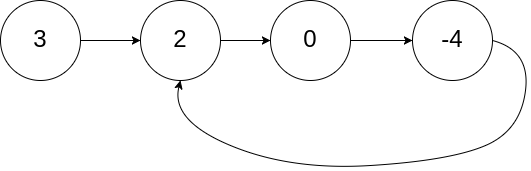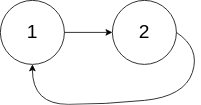# LinkedList - 142. Linked List Cycle II

1. Linked List Cycle II

Given a linked list, return the node where the cycle begins. If there is no cycle, return `null`.

To represent a cycle in the given linked list, we use an integer `pos` which represents the position (0-indexed) in the linked list where tail connects to. If `pos` is `-1`, then there is no cycle in the linked list.

Note: Do not modify the linked list.

Example 1:

Input: head = [3,2,0,-4], pos = 1
Output: tail connects to node index 1
Explanation: There is a cycle in the linked list, where tail connects to the second node.Example 2:

Input: head = [1,2], pos = 0
Output: tail connects to node index 0
Explanation: There is a cycle in the linked list, where tail connects to the first node.Example 3:

Input: head = , pos = -1
Output: no cycle
Explanation: There is no cycle in the linked list.Follow-up:
Can you solve it without using extra space?

``````详细解释一下推算过程：

L + k * S + (S - U) = 2(L + (S - U))

L = (k - 1) * S + U, (U <= S)

``````

java：

``````/**

* Definition for singly-linked list.
* class ListNode {
*     int val;
*     ListNode next;
*     ListNode(int x) {
*         val = x;
*         next = null;
*     }
* }
*/
public class Solution {
public ListNode detectCycle(ListNode head) {
if (head == null || head.next == null) return null;

ListNode slow = head;
ListNode fast = head;
while (fast != null && fast.next != null) {
slow = slow.next;
fast = fast.next.next;
if (fast == slow) {
while (head != fast) {
fast = fast.next;
head = head.next;
}
return head;
}
}

return null;
}
}
``````

go：

``````/**

* Definition for singly-linked list.
* type ListNode struct {
*     Val int
*     Next *ListNode
* }
*/
func detectCycle(head *ListNode) *ListNode {
var (
res *ListNode
slow = head
fast = head
)

if head == nil || head.Next == nil {
return res
}

for fast != nil && fast.Next != nil {
slow = slow.Next
fast = fast.Next.Next
if slow == fast {
fast = head
for slow != fast {
slow = slow.Next
fast = fast.Next
}
return slow
}
}

return res
}
``````

Nothing just happens, it's all part of a plan.